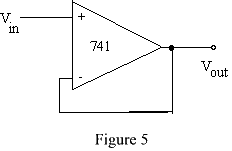EE 212 Lab
Lab 7: Operational Amplifiers - Part I

Data Sheet: National Semiconductor LM741 Operational Amplifier (Please don't print whole data sheet)

The purpose of this lab is to build and study basic amplifier circuits that use operational amplifiers (op-amps). The National Semiconductor LM741 (see data sheet link above) will be the op-amp used in this lab.

Notes on Measurements:

• Make all measurements carefully, i.e., they should be repeatable and accurate to within 5%. If you can't obtain this, ask for help.
• Adjust the scope for high accuracy - a waveform that takes up the full oscilloscope display can be measured much more accurately than one displayed on some fraction of the display.
• When asked if measurements agree with what is expected, do the necessary analytic calculations for comparison. Some calculations can be done before lab, and feel free to bring and use your class notes and book.

Laboratory Procedure:

1. LM741 specifications from data sheet
• Give the data sheet for the LM741 op-amp a quick review noticing all the associated specifications.
• Sketch the pin-out (connection diagram) for the LM741 you received in your packet with the graphical representation of the op-amp overlaid to show connections. Show this diagram to your lab instructor to make sure all the connections are understood before proceeding (wrong connections will likely damage the op-amp in a hurry).
• Note how to tell the location of pin 1 on the integrated circuit.
• Note the absolute maximum supply voltages that should be used to power the op-amp.
2. Inverting Amplifiers
• Wire up an inverting amplifier having an input resistance of 1kW and gain of -10 as shown in figure 1. Use a LM741 op-amp with supply voltages of plus and minus 15V. Employ good breadboard technique by using the bus lines on your breadboard for each of the supply voltages and ground.
• Vary the DC level of the op-amp input by turning the 10kW pot and take a couple of measurements to confirm that the amplifier is working as expected.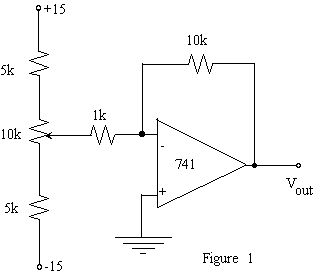• Add a 1kHz, 1Vp-p sine wave (shown as Vs) to the amplifier input as shown in figure 2.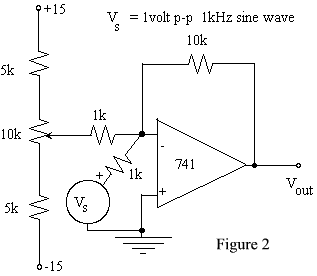• Measure the gain for several input amplitudes and compare with the theoretical gain.
• Confirm that the amplifier inverts the input by displaying both input and output sine waves on the scope (sketch waveforms).
• Vary the DC level of the input by turning the 10kW pot and confirm that the amplifier is summing the two inputs.
• Remove the DC input and apply only the 1kHz, 1Vp-p sinusoidal input through an additional 1kW resistor as shown in figure 3.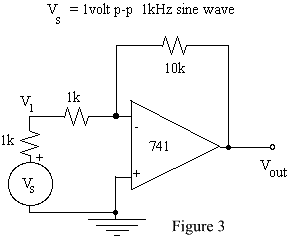• Calculate the amplifier circuit's input resistance looking into the amplifier circuit from the node labeled V1, at 1kHz, using the node voltages VS and V1. Question: Why use this size of resistor - why not a larger or smaller value?
• Measure (or try to) the output resistance of the amplifier circuit by representing it with a Thevenin equivalent circuit that can be found by putting a 1kW resistor from the output to ground and noting how much the output voltage changes. The change in the output voltage can be thought of as a voltage divider between Rout and the 1kW resistor. Question: Why use this size of resistor - why not a larger or smaller value?
• Increase the input amplitude to determine the voltage levels at which the output 'saturates'. How do these voltages compare to the supply voltages?
3. Non-Inverting Amplifier
• Wire up the non-inverting amplifier shown in figure 4. What is the amplifier's voltage gain? (Note: it's not the same as the last circuit.)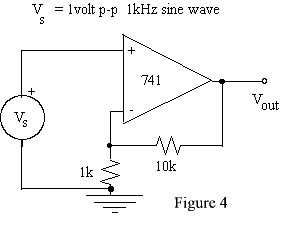• Measure its input resistance, at 1kHz, by putting a 100kW or 1MW resistor in series with the input VS. Question: Why use this size of resistor - why not a larger or smaller value?
• Does this configuration maintain the low output impedance you measured for the inverting amplifier?
4. Follower
• Build a follower with the 741 op-amp as shown in figure 5. Check its performance, in particular measure (if possible) Zin and Zout.